Skip to content

# marcomusy/vtkplotterFetching latest commit…
Cannot retrieve the latest commit at this time.
Type Name Latest commit message Commit time
..
Failed to load latest commit information.README.mdconvexHull.ipynbconvexHull.pycutAndCap.pycutWithMesh.pydensifycloud.pyfatlimb.pyfitplanes.pyfitspheres1.pyfitspheres2.pygeodesic.pyinterpolateField.pyinterpolateScalar.pymesh_smoothers.pymeshquality.pymoving_least_squares1D.pymoving_least_squares2D.pymoving_least_squares3D.pypointsCutMesh1.pypointsCutMesh2.pyprojectsphere.pyquadratic_morphing.pyrecosurface.pyskeletonize.pysplitmesh.pythinplate.pythinplate_grid.ipynbthinplate_grid.pythinplate_morphing.pythinplate_morphing_2d.py

# Advanced examples

In this directory you will find a set of examples to perform more complex operations and simulations.

```git clone https://github.com/marcomusy/vtkplotter.git
cd vtkplotter/examples/advanced
python example.py  # on mac OSX try 'pythonw' instead```

(click thumbnail image to get to the python script)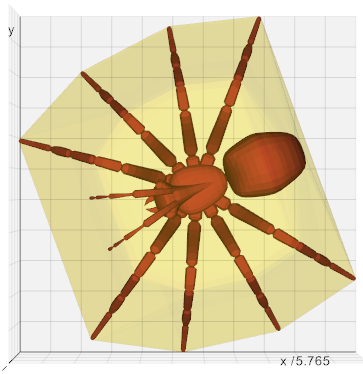`convexHull.py`
Create a 3D Delaunay triangulation of a set of input points.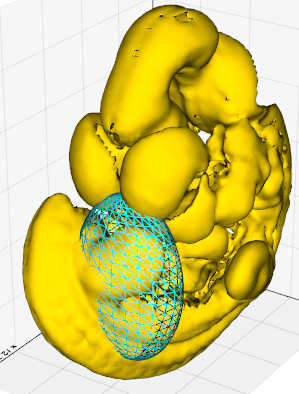`cutWithMesh.py`
Cut a mesh with another mesh.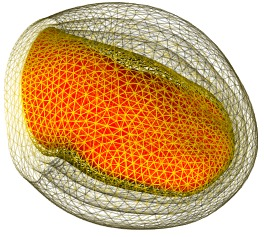`fatlimb.py`
Modify a mesh by moving the points along the normals to the surface and along the radius of a sphere centered at the center of mass of the mesh. At each step we redefine the actor so that the normals are recalculated for the underlying polydata.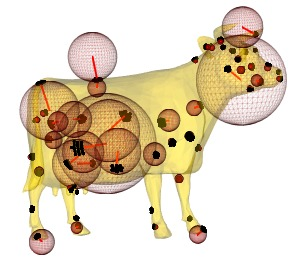`fitspheres1.py`
Fit spheres to a region of a surface defined by N points that are closest to a given point of the surface. For some of these points we show the fitting sphere.
Red lines join the center of the sphere to the surface point.
Black points are the N points used for fitting.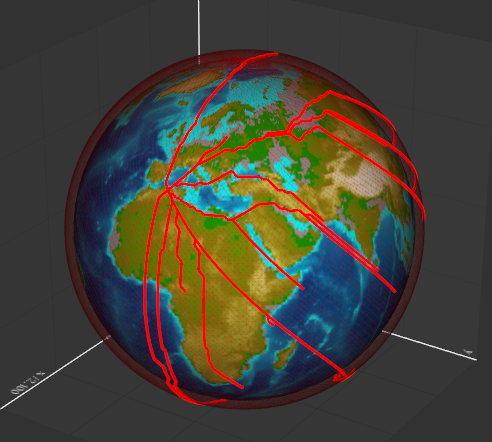`geodesic.py`
Dijkstra algorithm to compute the mesh geodesic shortest path.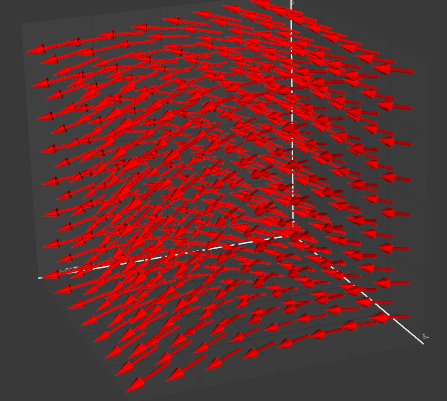`interpolateField.py`
Interpolate the value of a vectorial field only known on a small set of points to a whole region space where the field is not defined.
Two interpolation methods are shown: Radial Basis Function and Thin Plate Splines.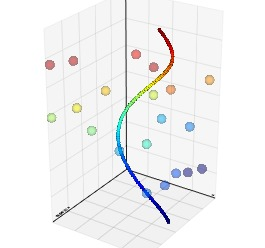`interpolateScalar.py`
Interpolate the value of a scalar only known on a set of points to a new set of points where the scalar is not defined.
Two interpolation methods are possible: Radial Basis Function and Nearest point.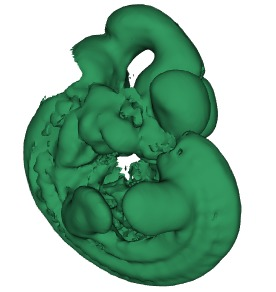`mesh_smoothers.py`
Mesh smoothing with `smoothLaplacian()` and `smoothWSinc()` methods.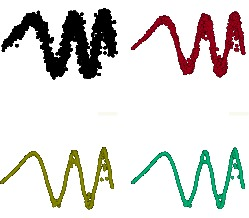`moving_least_squares1D.py`
Use a variant of the Moving Least Squares algorithm for a cloud of scattered points to become a smooth line. The input actor's polydata is modified by the method so more than one pass is possible.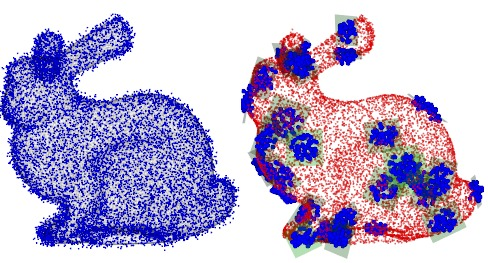`moving_least_squares2D.py`
Use a variant of the Moving Least Squares algorithm for a cloud of points to become a smooth surface. The input actor's polydata is modified by the method so more than one pass is possible.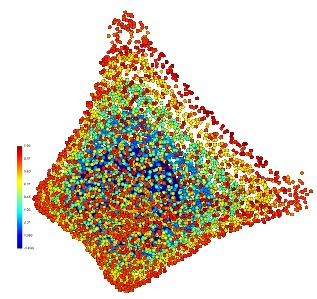`moving_least_squares3D.py`
Generate a time sequence of 3D shapes (from a blue sphere to a red tetrahedron) as noisy cloud points, and smooth it with Moving Least Squares. This make a simultaneus fit in 4D (space+time).
`smoothMLS3D()` method returns an actor where points are color coded in bins of fitted time.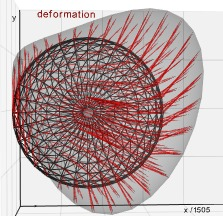`quadratic_morphing.py`
Takes two meshes, source and target, and morphs source to target. This is obtained by fitting 18 parameters of a non linear, quadratic, transformation defined in `transform()`. The fitting minimizes the distance to the target surface.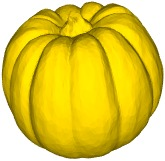`recosurface.py`
Reconstruct a surface from a point cloud.
1. An mesh is loaded and noise is added to its vertices.
2. the point cloud is smoothened with MLS (see `moving_least_squares2D.py`)
3. `mesh.clean()` imposes a minimum distance among mesh points.
4. a triangular mesh is extracted from this smoother point cloud.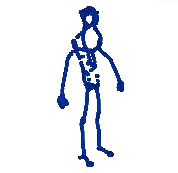`skeletonize.py`
Using 1D Moving Least Squares to skeletonize a surface.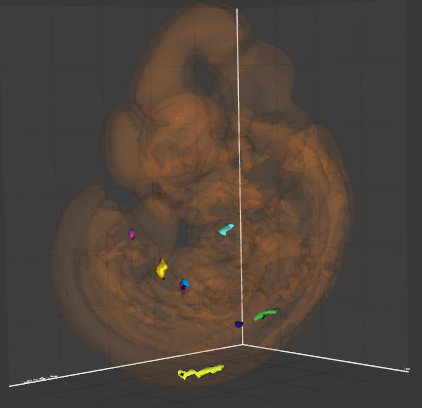`splitmesh.py`
Split a mesh by connectivity and order the pieces by increasing value of the area.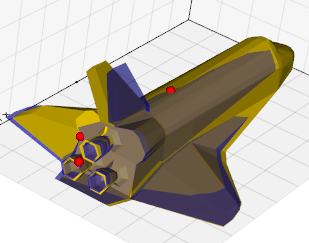`thinplate.py`
Perform a nonlinear warp transformation defined by a set of source and target landmarks.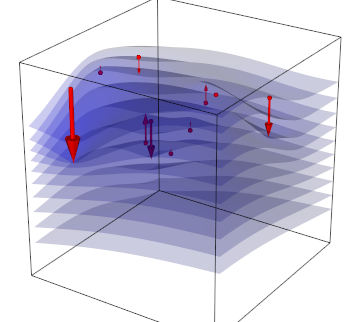`thinplate_grid.py`
Move a set of control points to warp space in their vicinity. Warping is visualised through a set of horizontal grids.
You can’t perform that action at this time.